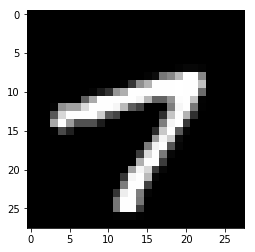# Overview of Keras/TensorFlow Basic Operations

I in the process of updating my deep learning course and books to make use of Keras. This posting contains some of the basic examples that I put together. This post is not meant to be an introduction to neural networks in general. For such an introduction, refer to either my books or this article.

I will expand on these examples greatly for both the book and course. The basic neural network operations that I needed were:

• Simple Regression
• Regression Early Stopping
• Simple Classification
• Classification Early Stopping
• Deep Neural Networks w/Dropout and Other Regularization
• Convolutional Neural Networks
• LSTM Neural Networks

These are some of the most basic operations that I need to perform when working with a new neural network package. This provides me with a sort of Rosetta Stone for a new neural network package. Once I have these operations, I can more easily create additional examples that are more complex.

The first thing to check is what versions you have of the required packages:

The following functions are from my set of helpful functions that I created for my class and use in many of my books:

## Simple Regression

Regression is where a neural network accepts several values (predictors) and produces a prediction that is numeric. In this simple example we attempt to predict the miles per gallon (MPG) of several cars based on characteristics of those cars. Several parameters and used below and described here.

• Losses Supported by Keras
• Typically use mean_squared_error for regression (the square root of mean square error is root mean square error(RMSE)).
• and for classification use: binary_crossentropy for 2 classes, categorical_crossentropy for more than 2 classes.
• kernel_initializer supported by Keras - Species how the weights of are randomized.
• activation - Usually relu or softmax will be used.

Now that the neural network is trained, we will test how good it is and perform some sample predictions.

## Regression (Early Stop)

Early stopping sets aside a part of the data to be used to validate the neural
network. The neural network is trained with the training data and validated
with the validation data. Once the error no longer improves on the validation
set, the training stops. This prevents the neural network from overfitting.

## Classification Model (Early Stop)

Early stopping can also be used with classification. Early stopping sets aside a part of the data to be used to validate the neural network. The neural network is trained with the training data and validated with the validation data. Once the error no longer improves on the validation set, the training stops. This prevents the neural network from overfitting.

Show the predictions (raw, probability of each class.)

## The Classic MNIST Dataset

The next examples will use the MNIST digits dataset. The previous examples used CSV files to load training data. Most neural network frameworks, such as Keras, have common training sets built in. This makes it easy to run the example, but hard to abstract the example to your own data. Your on data are not likely built into Keras. However, the MNIST data is complex enough that it is beyond the scope of this article to discuss how to load it. We will use the MNIST data build into Keras.## Convolutional Neural Networks

Convolutional Neural Networks are specifically for images. They have been applied to other cases; however, use beyond images is somewhat rarer than with images.

## Long Short Term Memory (LSTM)

Long Short Term Memory is typically used for either time series or natural language processing (which can be thought of as a special case of natural language processing).